# Math in Focus Grade 5 Chapter 8 Practice 2 Answer Key Comparing and Rounding Decimals

Practice the problems of Math in Focus Grade 5 Workbook Answer Key Chapter 8 Practice 2 Comparing and Rounding Decimals to score better marks in the exam.

## Math in Focus Grade 5 Chapter 8 Practice 2 Answer Key Comparing and Rounding Decimals

Compare the decimals in each place-value chart.
Fill in the blanks. Write > or < in the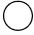.

Example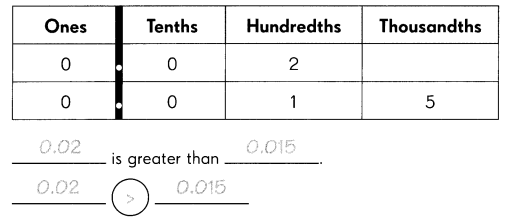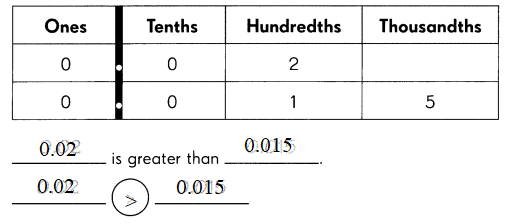Question 1.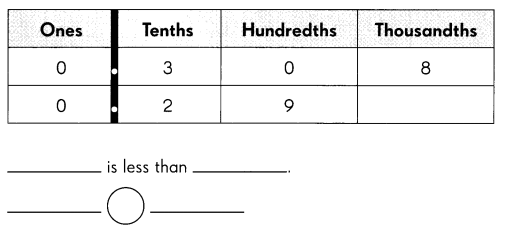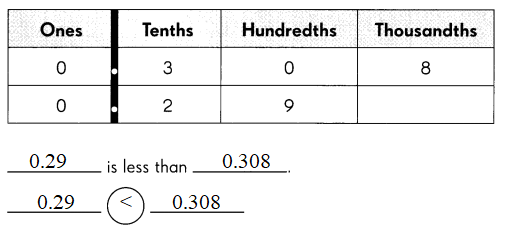0.29 less than 0.308
0.29 < 0.308

Question 2.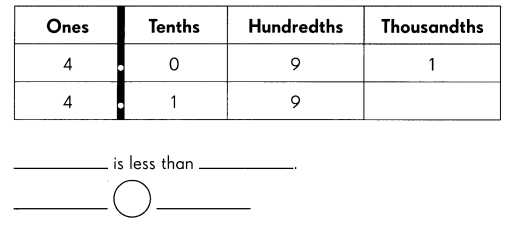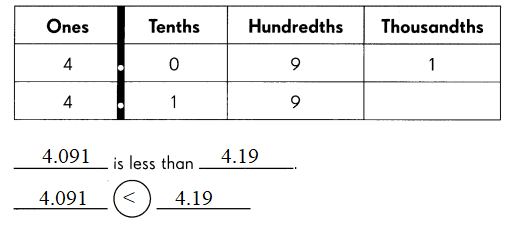4.091 is less than 4.19
4.091 < 4.19

Write the greater decimal.

Question 3.
11.6 or 21.8 _____
The greatest decimal is 21.8.

Question 4.
10.55 or 10.05 _____
The greatest number is 10.55.

Question 5.
20.07 or 20.01 _____
The greatest number is 20.07.

Question 6.
100.202 or 100.212 _____
The greatest number is 100.212.

Write >, <,or = in each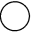.

Question 7.
3.70.370
3.7 > 0.370

Question 8.
0.1500.51
0.150 < 0.51

Question 9.
0.2052.05
0.205 < 2.05

Question 10.
2.32.30
2.3 = 2.30

Circle the greatest decimal and underline the least.

Question 11.
1.03, 1.3, 0.13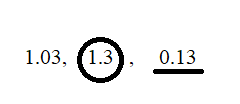1.3 is the greatest decimal number.
0.13 is the least decimal number.

Question 12.
0.5, 0.53, 0.503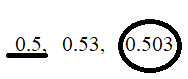0.503 is the greatest decimal number.
0.5 is the least decimal number.

Question 13.
2.35, 2.305, 2.035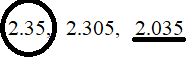2.35 is the greatest decimal number.
2.035 least decimal number.

Question 14.
8.7, 8.07, 8.701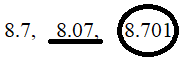8.701 is the greatest decimal number.
8.07 least decimal number.

Order the decimals from least to greatest.

Example
3.33, 3.03, 3.303 3.03, 3.303, 3.33

Question 15.
5.51, 5.051, 5.501 _____
The given decimal numbers from least to greatest numbers are 5.051, 5.501, 5.51.

Question 16.
4, 4.01. 4.001 ______
The given decimal numbers from least to greatest number are 4, 4.001, 4.01.

Question 17.
0.023, 0.203, 0.230 __________
The given decimal number from least to greatest number are 0.023, 0.203, 0.230.

Write the missing decimal in each box. Round the given decimal to the nearest hundredth.

Question 18.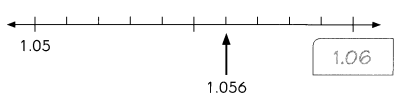1 .056 rounded to the nearest hundredth is ___________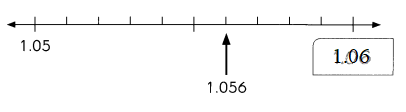1.056 rounded to the nearest hundredth is 1.06.

Question 19.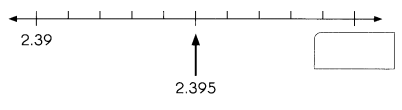2.395 rounded to the nearest hundredth is ___.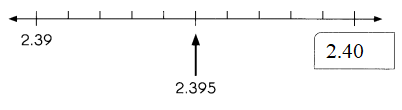2.395 rounded to the nearest hundredth is 2.40.

Question 20.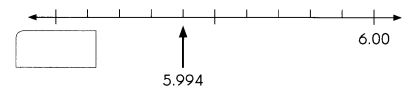5.994 rounded to the nearest hundredth is ___.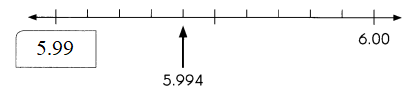5.994 rounded to the nearest hundredth is 5.99

Fill in the blanks.

Question 21.
The mass of a sewing needle is 0.585 gram. Round the mass to the nearest hundredth of a gram. __________ rounds to ________________ .
0.585 rounds to 0.59 grams.

Question 22.
The width of a pinhead is 0.098 centimeter. Round the width to two decimal places. ________________ rounds to ________________.
0.098 rounds to 0.10 cm.

Question 23.
1 centimeter is equal to 0.394 inches. Round 0.394 inches to the nearest hundredth of an inch. ________________ rounds to ________________ .
0.394 rounds to 0.39 inches.

Round each decimal to the nearest whole number, nearest tenth, and nearest hundredth.

Question 24.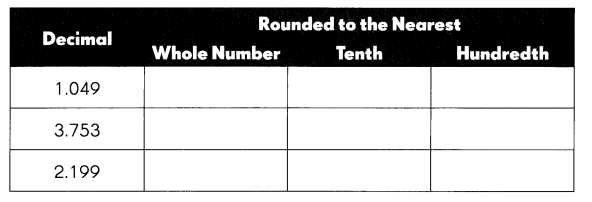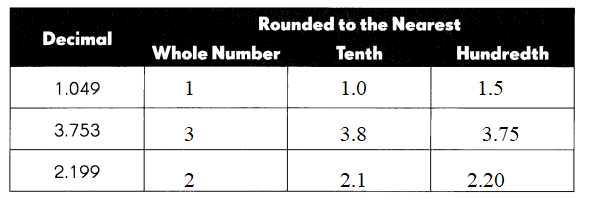For 1.049 whole number is 1, tenth number is 1.0, hundredth number is 1.05.
For 3.753 whole number is 3, tenth number is 3.8, hundredth number is 3.75.
For 2.199 whole number is 2, tenth number is 2.1, hundredth number is 2.20

Fill in the blanks.

Question 25.
A decimal rounded to the nearest tenth is 2.5. Write two decimals that can be rounded to 2.5.
____ and _____
Two decimals that can be rounded to 2.5 are 2.49 and 2.51.

Question 26.
A decimal rounded to the nearest hundredth is 4.09. Write two decimals that can be rounded to 4.09.
____ and _____